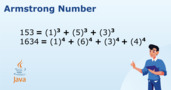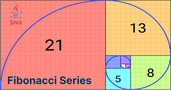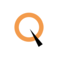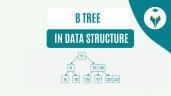How to Find Reverse of a Number in Java

# How to Find Reverse of a Number in Java

clickHere
Updated on Jan 10, 2023 16:55 IST

Reversing a number in Java is a common task that can be accomplished using a variety of methods. For example, you can use a loop to iterate over the digits of the number and build the reversed number one digit at a time. Alternatively, you can convert the number to a string, reverse the string using the built-in StringBuilder class, and then parse the reversed string back into a number. Other options include using a character array or recursion to reverse the number. When deciding on the best method, consider factors such as time complexity, space complexity, and code simplicity.

In this blog we will learn 5 different ways in which you can write a function to find the reverse of a number in Java.

Problem: Write a Java program that reads an integer from the user and prints the reverse of the integer. The program should continue to prompt the user for integers until the user enters 0.

Example:

```Enter a number: 12345
The reverse of 12345 is 54321
Enter a number: 123
The reverse of 123 is 321
Enter a number: 0
Cannot reverse a single digit number```

Explore other popular Java Courses

## Method 1: Reverse a Number in Java Using a Loop

• Initialize a variable reverse to 0.
• While num is not 0:
• Append the last digit of num to reverse by performing the following steps:
• Multiply reverse by 10.
• Add the last digit of num to reverse.
• Remove the last digit of num by dividing num by 10.
• Return reverse.
` `
`public static int reverse(int num) {    int reverse = 0;    while (num != 0) {        reverse = reverse * 10 + num % 10;        num /= 10;    }    return reverse;}Copy code`

### Dry Run Explanation:

Let’s say we want to reverse the number 12345. Here is how the method would work:

Read more: Reverse a String in JavaArmstrong Number in Java using Different Methods
Learn to check if a number is an Armstrong number in Java using while loop or recursion. Understand the mathematical definition and see code examples for easy implementationCheck Palindrome in Java Using Different Methods
Discover various methods for checking if a string is a palindrome in Java. Use recursion and string manipulation to efficiently determine if a string is a palindrome, ignoring spaces, punctuation,...read moreFibonacci Series in Java [Case-Study Based]
The Fibonacci series is a sequence of numbers in which each number is the sum of the two preceding ones, usually starting with 0 and 1. In Java, the Fibonacci...read more

## Method 2: Reverse a Number in Java Using a String

• Convert num to a string.
• Initialize a variable reverse to an empty string.
• Iterate through the characters of the string in reverse order:
• Append each character to reverse.
• Convert reverse back to an integer and return it.
` `
`public static int reverse(int num) {    String str = Integer.toString(num);    String reverse = "";    for (int i = str.length() - 1; i >= 0; i--) {        reverse += str.charAt(i);    }    return Integer.parseInt(reverse);}Copy code`

### Dry Run Explanation:

Let’s say we want to reverse the number 12345. Here is how the method would work:Swapping of Two Numbers in Java
Get an introduction to swapping the values of two numbers in Java using different methods. Compare the use of a temporary variable, arithmetic operators, and bitwise operators for swapping numbers.How to Check Leap Year in Java?
In this blog, you will learn how to check leap year in Java. A leap year is a year that is divisible by 4 and 400 but not by 100.Switch Case in Java with Examples
Switch statements in Java enable execution of different code blocks based on the value of an expression. They provide an alternative to multiple if-else statements and can be used when...read more

## Method 3: Reverse a Number in Java Using an Array

• Convert num to a string and then to an array of characters.
• Initialize an array reverse of the same size as the original array.
• Iterate through the original array in reverse order:
• Set the corresponding element of reverse to the current element of the original array.
• Convert reverse back to a string and then to an integer and return it.
` `
`public static int reverse(int num) {    char[] arr = Integer.toString(num).toCharArray();    char[] reverse = new char[arr.length];    for (int i = 0; i < arr.length; i++) {        reverse[i] = arr[arr.length - i - 1];    }    return Integer.parseInt(new String(reverse));}Copy code`

### Dry Run Explanation:

Let’s say we want to reverse the number 12345. Here is how the method would work:

In the last line of code, the reverse char array is being converted back to a string using the new String(reverse) constructor. This creates a new string object that contains the same sequence of characters as the reverse array.

Then, the Integer.parseInt() method is being called on the string to parse it as an integer. This returns an int value that is the integer representation of the string. Finally, this int value is returned as the output of the reverse() method.

## Method 4: Reverse a Number in Java Using Recursion

• If num is less than 10, return num.
• Otherwise, return the last digit of num concatenated with a recursive call to reverse the rest of num.
` `
`public static int reverse(int num) {    if (num < 10) {        return num;    }    return Integer.parseInt(num % 10 + "" + reverse(num / 10));}Copy code`

### Dry Run Explanation:

Let’s say we want to reverse the number 12345. Here is how the method would work:

## Time and Space Complexity Comparison

In terms of time complexity, the fourth method (using recursion) has the lowest time complexity, followed by the first method (using a loop). The second and third methods (using a string and a character array, respectively) have the same time complexity.

In terms of space complexity, the first method (using a loop) has the lowest space complexity, followed by the second and third methods (using a string and a character array, respectively). The fourth method (using recursion) has the highest space complexity.

clickHere

Experienced AI and Machine Learning content creator with a passion for using data to solve real-world challenges. I specialize in Python, SQL, NLP, and Data Visualization. My goal is to make data science engaging an... Read Full Bio

## Trending Technology CoursesProgramming in Java by NPTEL
NPTEL4.6Java Full Stack
Qspider4.6## Top Picks & New Arrivals